Question

Consider an RC circuit in which a capacitor is being charged by a battery connected in a circuit. Initially the capacitor is uncharged. Then the switch is closed. After a time equal to 2.9 time constants, what percent of the final charge is present on the capacitor? Answer in units of %.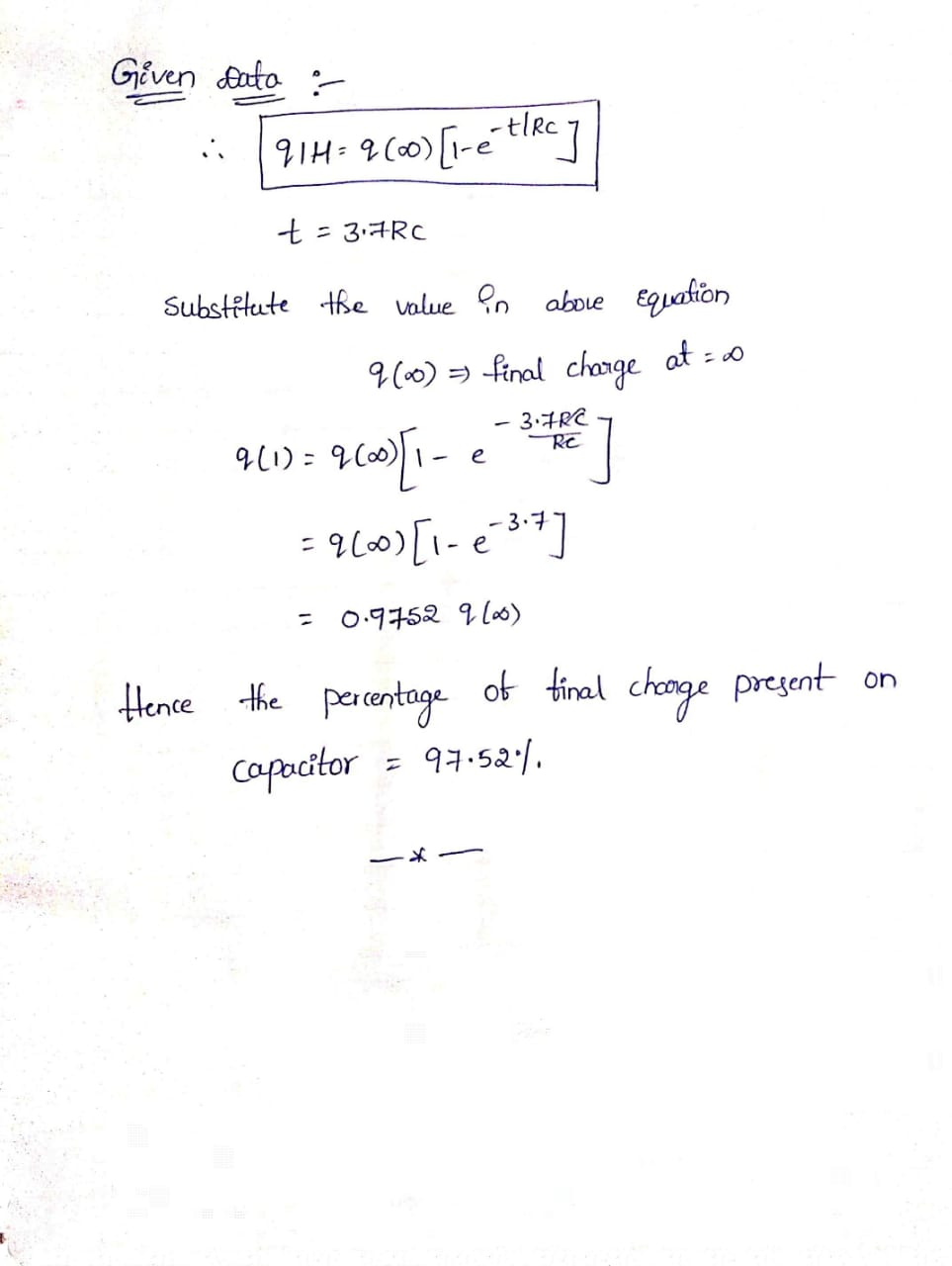#### Earn Coins

Coins can be redeemed for fabulous gifts.

Similar Homework Help Questions
• ### HE In the given RC circuit, a capacitor is connected to a resistor in series and...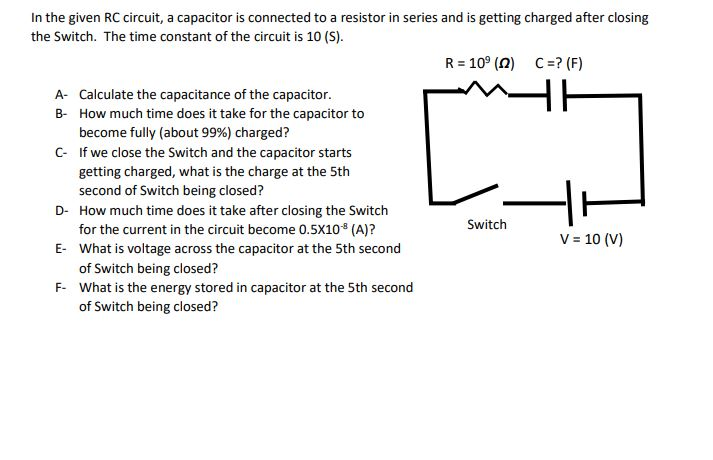HE In the given RC circuit, a capacitor is connected to a resistor in series and is getting charged after closing the Switch. The time constant of the circuit is 10 (s). R = 109 (0) C =? (F) A- Calculate the capacitance of the capacitor. B- How much time does it take for the capacitor to become fully (about 99%) charged? C- If we close the Switch and the capacitor starts getting charged, what is the charge at the...

• ### HA In the given RC circuit, a capacitor is connected to a resistor in series and...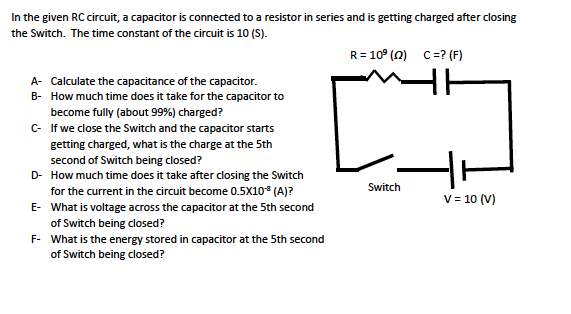HA In the given RC circuit, a capacitor is connected to a resistor in series and is getting charged after closing the Switch. The time constant of the circuit is 10 (S). R = 10° (0) C =? (F) A- Calculate the capacitance of the capacitor. B- How much time does it take for the capacitor to become fully (about 99%) charged? C- If we close the Switch and the capacitor starts getting charged, what is the charge at the...

• ### Problem 2 HE In the given RC circuit, a capacitor is connected to a resistor in...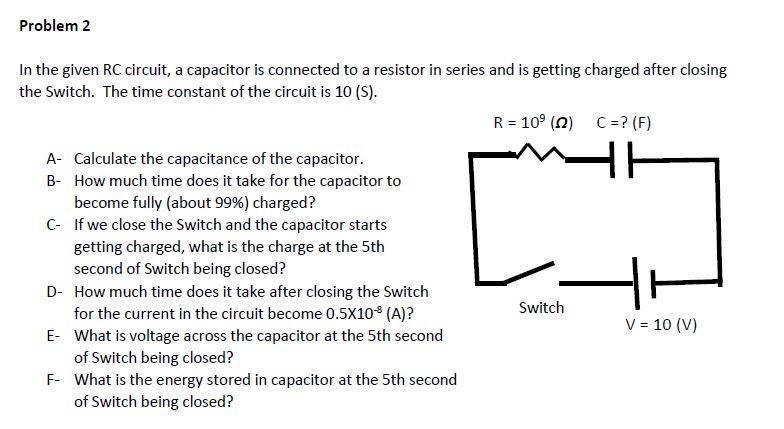Problem 2 HE In the given RC circuit, a capacitor is connected to a resistor in series and is getting charged after closing the Switch. The time constant of the circuit is 10 (S). R = 10º (2) C=? (F) A- Calculate the capacitance of the capacitor. B- How much time does it take for the capacitor to become fully (about 99%) charged? C- If we close the Switch and the capacitor starts getting charged, what is the charge at...

• ### Problem 2 HE In the given RC circuit, a capacitor is connected to a resistor in...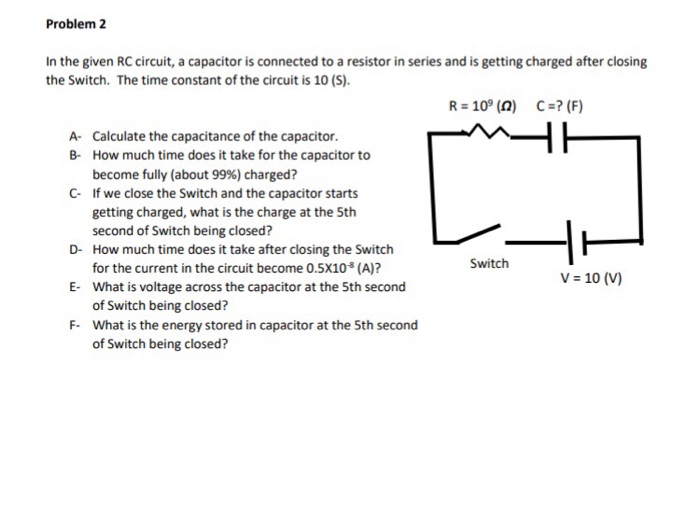Problem 2 HE In the given RC circuit, a capacitor is connected to a resistor in series and is getting charged after closing the Switch. The time constant of the circuit is 10 (s). R = 10° (0) C=? (F) A- Calculate the capacitance of the capacitor. B- How much time does it take for the capacitor to become fully (about 99%) charged? C- If we close the Switch and the capacitor starts getting charged, what is the charge at...

• ### Problem 2 In the given RC circuit, a capacitor is connected to a resistor in series...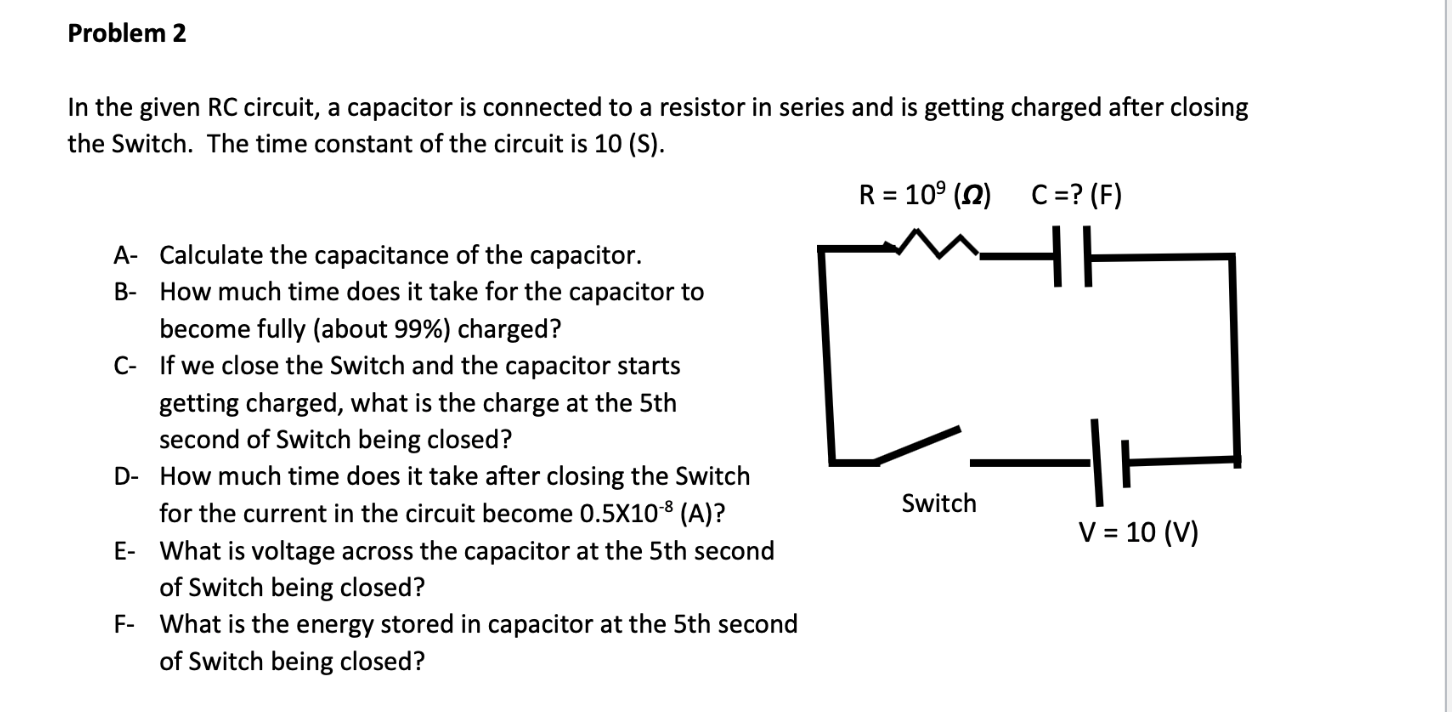Problem 2 In the given RC circuit, a capacitor is connected to a resistor in series and is getting charged after closing the Switch. The time constant of the circuit is 10 (S). R = 109 (2) C =? (F) A- Calculate the capacitance of the capacitor. B- How much time does it take for the capacitor to become fully (about 99%) charged? C- If we close the Switch and the capacitor starts getting charged, what is the charge at...

• ### Problem 2 HE In the given RC circuit, a capacitor is connected to a resistor in...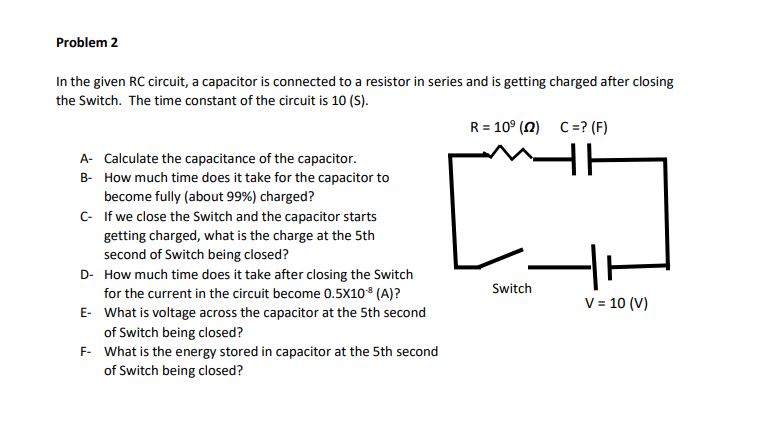Problem 2 HE In the given RC circuit, a capacitor is connected to a resistor in series and is getting charged after closing the Switch. The time constant of the circuit is 10 (S). R = 109 (0) C =? (F) A- Calculate the capacitance of the capacitor. B- How much time does it take for the capacitor to become fully (about 99%) charged? C- If we close the Switch and the capacitor starts getting charged, what is the charge...

• ### Problem 2 mA In the given RC circuit, a capacitor is connected to a resistor in...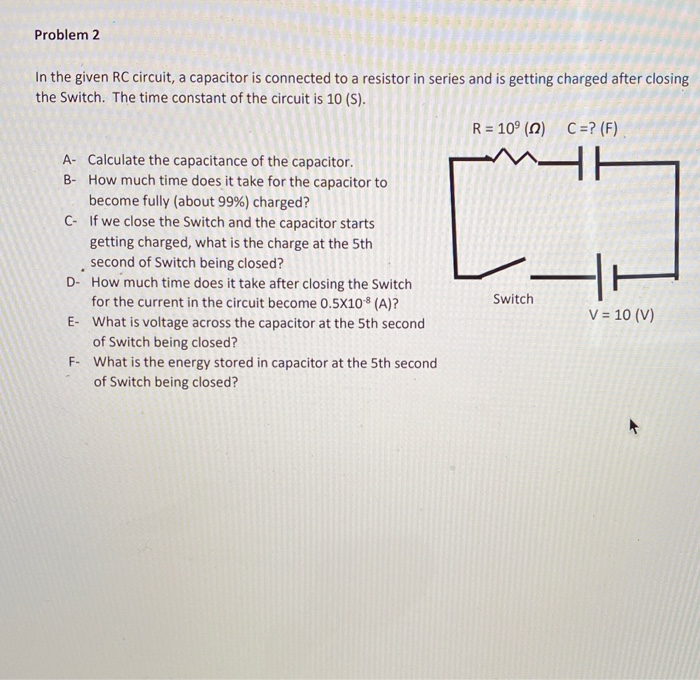Problem 2 mA In the given RC circuit, a capacitor is connected to a resistor in series and is getting charged after closing the Switch. The time constant of the circuit is 10 (S). R = 109 (0) C =? (F) A- Calculate the capacitance of the capacitor. B- How much time does it take for the capacitor to become fully (about 99%) charged? C- If we close the Switch and the capacitor starts getting charged, what is the charge...

• ### please answer asap Problem 2 In the given RC circuit, a capacitor is connected to a...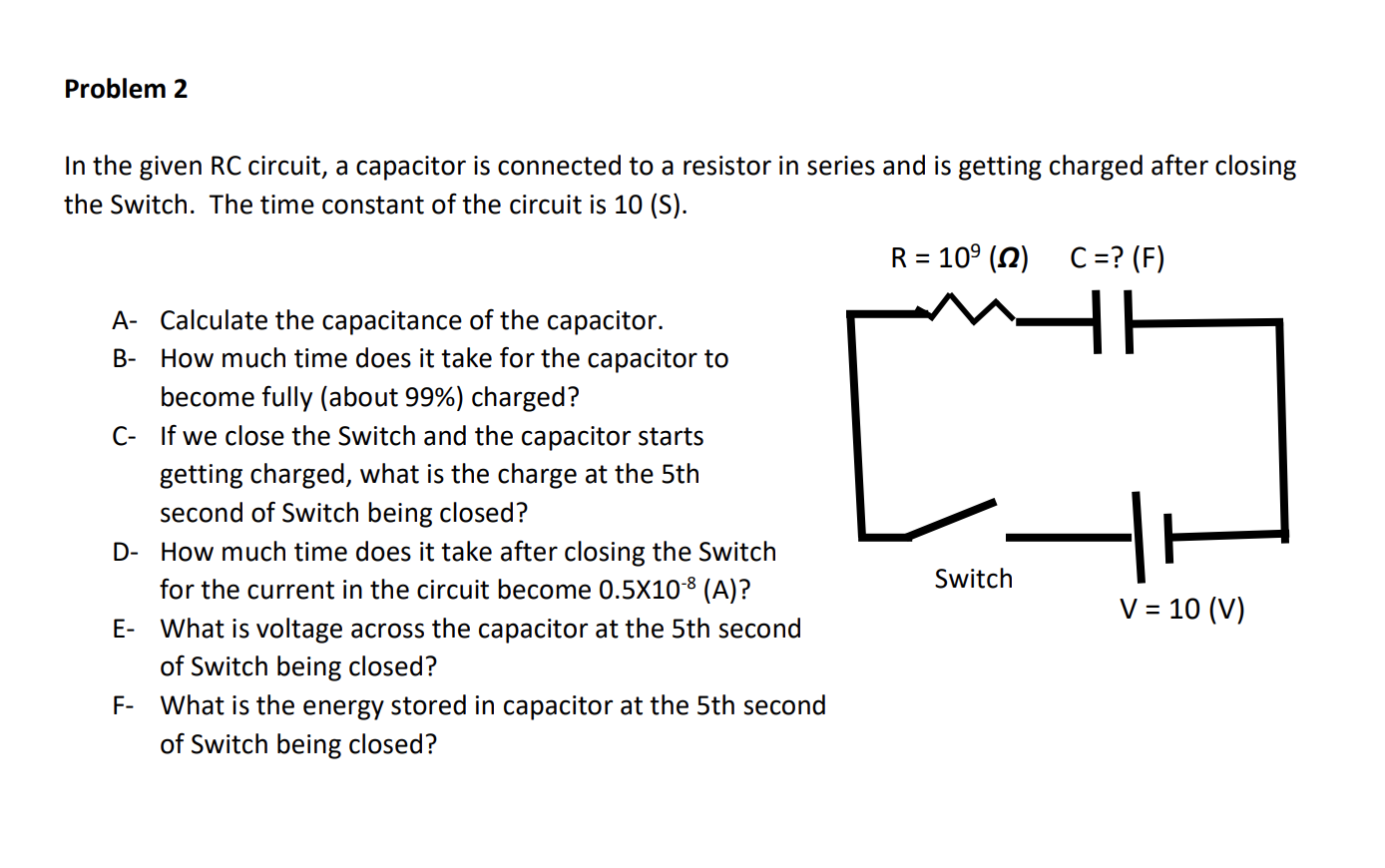please answer asap Problem 2 In the given RC circuit, a capacitor is connected to a resistor in series and is getting charged after closing the Switch. The time constant of the circuit is 10 (S). R = 109 (12) C =? (F) O A- Calculate the capacitance of the capacitor. B- How much time does it take for the capacitor to become fully (about 99%) charged? C- If we close the Switch and the capacitor starts getting charged, what...

• ### Consider the RC circuit in the figure. The switch has been open for a long time...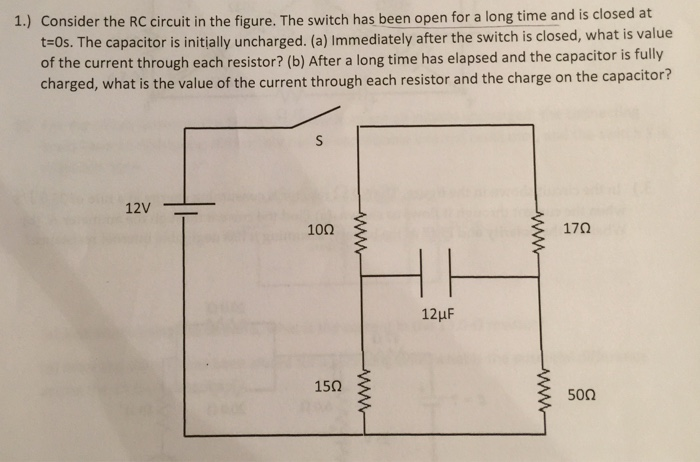Consider the RC circuit in the figure. The switch has been open for a long time and is closed at t=0s. The capacitor initially uncharged. (a) Immediately after the switch is closed, what is value of the current through each resistor? (b) After a long time has elapsed and the capacitor is fully charged, what is the value of the current through each resistor and the charge on the capacitor?

• ### Power in RC Circuits- RC-9 A battery with &-15.0V is connected in series with a resistor...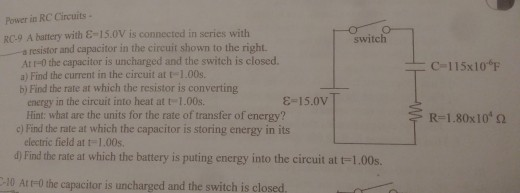Power in RC Circuits- RC-9 A battery with &-15.0V is connected in series with a resistor and capacitor in the circuit shown to the right. At r-0 the capacitor is uncharged and the switch is closed. a) Find the current in the circuit at t-1.00s. b) Find the rate at which the resistor is converting energy in the circuit into heat at t-1.00s. Hint: what are the units for the rate of transfer of energy? c) Find the rate at...